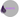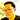# Pattern Matching in Elixir

December 19, 2017## Pattern Matching in Elixir

Pattern matching is a phrase you hear thrown around a lot as you get started in Elixir. But what is it exactly and how do you use it to write elegant code?

If you start to see the following use of “cond” blocks in your code, it’s a sign you might need some pattern matching. The below code shows signs of needing some pattern matching.

``````def compare(a, b) do
cond do
a === b ->
:equal
sublist?(a, b) ->
:sublist
sublist?(b, a) ->
:superlist
true ->
:unequal
end
end``````

### = is the first thing to understand

The first thing to understand is that the equals symbol (“=”) is a match operator. If you come from an object oriented language like Ruby, you may think of it as an “assignment operator”, but that’s not how it’s referred to in Elixir.

### Pattern matching with recursion

The other neat thing about Elixir is that it allows you to pattern match on a list using recursion. Perhaps it’s best to start with a code sample.

``````defmodule Calc do
end
def triple([]), do: []
end``````
``````iex> Calc.triple([2,4,6])
[6, 12, 18]``````

The triple function triples each element in a list. You’ll notice it’s done by “pattern matching” on the head of a list and then calculating the triple of the tail in the above code example.

### Using the _ operator to match anything

A lot of times in Elixir code, you’ll get back the status of some function call. For example, if successful, the following code returns something like {:ok, #<pid0.91.0>}.

For example, the code below does exactly that if successful.

`{_, pid} = Supervisor.start_child(__MODULE__, [id])`

### Pattern Matching Through Functional Arity

Now in Elixir, you can’t match by data type because it’s a dynamically typed language. So one way you can pattern match is by functional arity. Functional arity refers to the number of arguments in a function.

Thus, in the below code, you can call sum with 2 or 3 numerical arguments and still get back a correct result. This is thanks to pattern matching.

``````def sum(a, b, c), do: a + b + c
def sum(a, b), do: a + b``````

### Pattern Matching By Exact Values

The other thing I’ve done is pattern match by exact value. Here’s an example:

``````def operation(true, a, b), do: a + b
def operation(false, a, b), do: a - b``````

So now depending on the boolean value I pass into the first argument of the operation function, I get back either the sum or difference of the values of a and b.

### Pinning a variable

Recently, I learned about pinning variables. Sometimes we don’t want to use the “=” operator to rebind a value to a variable. Thus, we can use the pin operator to pattern match on the existing value of the variable. Here’s a code example to show what this means in practice.

We bind a value of 5 to x, then try to pattern match on 6. We get a MatchError.

``````x = 5
^x = 6

** (MatchError) no match of right hand side value: 6``````

### The order of functions matters

If in an Elixir code base I had function 1 above function 2, I would never actually get to function 2 if a token were nil. That’s because the order in which functions are defined in Elixir matters.

``````def ops(%{part: "snippets", token: token}), do: IO.puts "non nil"  #function 1
def ops(%{part: "snippets", token: nil}), do: IO.puts "nil"  #function 2``````

So if I were worried about getting burned by a nil token, I’d need to swap the order of function 1 and 2 above.

### You can use guards too

If I had a guard via the “when” clause checking if token was nil, then I could leave function 1 above function 2 and achieve the desired outcome.

``````def ops(%{part: "snippets", token: token}) when is_nil(token), do: IO.puts "non nil"  #function 1
def ops(%{part: "snippets", token: token}), do: IO.puts "nil"  #function 2``````

### Summary

And there you go. The above cases are some ways you’ll pattern match in Elixir.Written by Bruce Park who lives and works on the Internet building useful and not-so-useful things. He is sometimes around on Twitter.